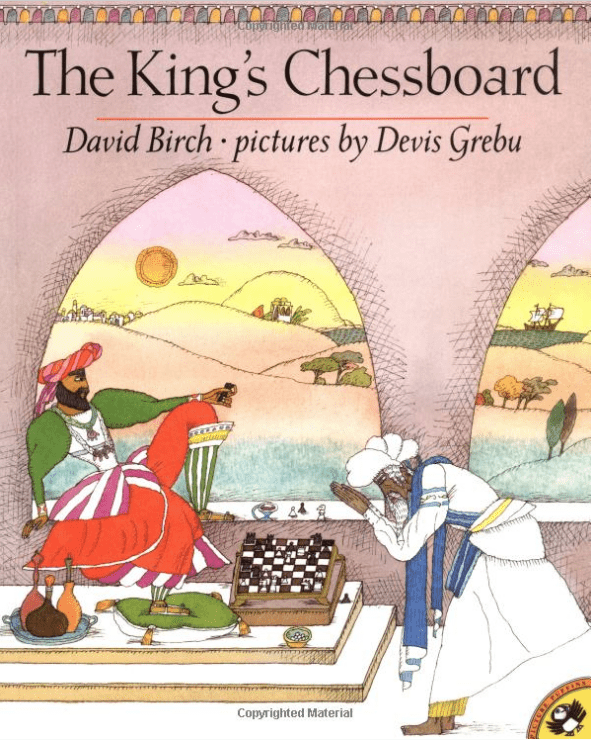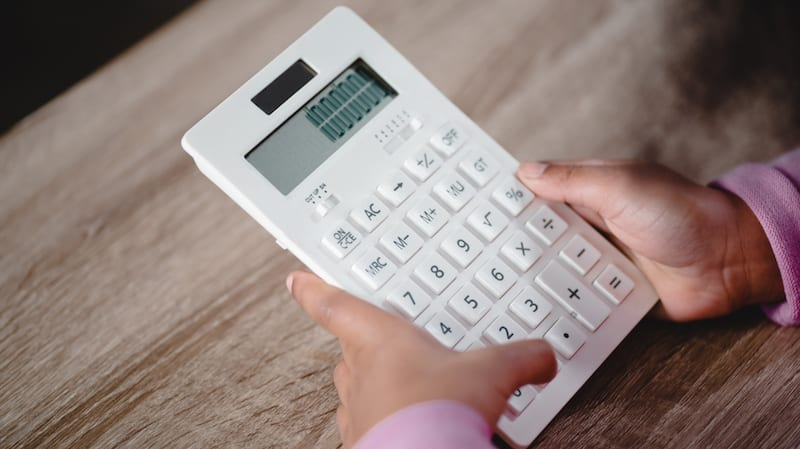Home » 9 Ways to Promote Algebraic Thinking in the Early Grades

# 9 Ways to Promote Algebraic Thinking in the Early Grades

It’s never too early to begin laying the foundations of algebraic thinking for young learners by helping them explore different concepts through simple, fun, but thought-stimulating activities.

Here are nine ways to cultivate algebraic thinking in young students.

Top ? credit: fantasticallyfourth on Instagram

## 1. Pattern Hunters

Much of math, and especially algebra, is based on patterns. Help young learners begin looking for patterns all around them. A great place to look is in the clothing we wear. Have students partner up and describe a pattern in their partner’s clothes, for example a striped shirt with blue and white alternating stripes. Have students draw a picture of the shirt to record the pattern.

## 2. The Pattern Museum

Provide students with manipulatives, like pattern blocks, buttons, keys, colored cubes, etc. Ask them to create two patterns on their desks with these materials. Classmates come around to visit the “pattern museum exhibits,” try to guess the patterns, and tell what would be next in the sequence.

## 3. Repeating Color Patterns

Some patterns repeat, such as X, O, X, O, X, O. Give students crayons and a piece of drawing paper. Ask them to choose two colors and to draw a repeating pattern of colored shapes, such as green triangle, blue circle, green triangle, blue circle. Partner students and have them see if they can extend their partner’s pattern by drawing in the element that would come next.

## 4. Match a Pattern

Partner students and provide them with a set of small objects and manipulatives. Ask one partner to be the leader. The leader takes some of the objects and creates a pattern on the desk, such as: penny, dime, nickel, penny, dime, nickel. The second partner then takes different objects and creates a matching pattern, such as: marble, key, button, marble, key, button. Both are ABC patterns but feature different materials. Have students take turns being the leader.

## 5. Growing Patterns Read AloudRead aloud The King’s Chessboard by David Birch. The King wants to reward a faithful wise man. At the king’s insistence, the wise man replies, “I only ask this: Tomorrow, for the first square of your chessboard, give me one grain of rice; the next day, for the second square, two grains of rice … and so on for every square on the chessboard.” The king agrees and soon learns a surprising and valuable lesson in mathematical growing patterns and humility, as the amount of rice quickly becomes more than all the rice in the world.

## 6. Function Machine

Functions are a big part of algebra. Help students learn about this idea with a function machine. Take a shoebox and cut a slot on the left side and a slot on the right side. Inside the box have a set of number cards, from 0–100. Give a student another set of identical number cards. Have the student choose a number and insert it on the left side. This is the input. You decide what the function of the box is. It could be + 2, – 1, whatever you wish. Let’s say the student puts in a 4. You decided your function is + 2. 4 + 2 = 6, so pick up a 6 and slide it out the output slot. Have three different students give you three different numbers and continue to add 2 to each number put in. Ask students if they can guess the machine’s function.

## 7. Calculator FunShow students how to turn a simple calculator into a function machine. Let’s say the function is + 2. Have students press “+,” then “2,” then “=.” From now on, every time students press “=” their calculator will add 2 to the previous number. Students can program their calculators with a function and see if classmates can guess it by viewing the outputs.

## 8. Mysterious X Number Riddles

In algebra, X is often used to represent an unknown. Young students can begin to work with X (or any other symbol for an unknown) in this way. Present students with three number sentences where X represents the same unknown and have them figure out what the value of X is. Introduce it as a number riddle. Give three clues to help students figure out what mysterious X is. For example: X + 1 = 3, X + 0 = 2, and 4 – X = 2. What is X? (X is 2.)

## 9. The Equal Sign = The Same As

Emphasize to students that when they are reading number sentences, and later algebraic equations, that the equal sign means “the same as.” Young children often think “=” means “the answer is.” For example, the equation 4 + 4 = 2 x 4 can be read as “four plus four is the same as two times four.” This will reinforce the idea of balancing equations because each side has the same quantity. For a simpler example, we could use 4 + 4 = 8 by saying “4 + 4 is the same as 8.” Also try placing the equal sign at the beginning of a number sentence, such as 8 = 4 + 4. This will help open up student understanding to what the equal sign is really all about.

How do you promote algebraic thinking in the early grades? Come and share in our WeAreTeachers HELPLINE group on Facebook.

Plus, check out our list of the best math websites.

0 comment